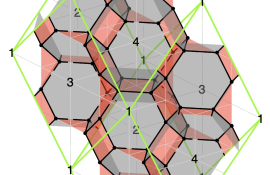### Mathematical Physics and AI Lab

수리물리학 및 AI 연구실I am doing research at the interface of mathematics and theoretical physics, with a particular emphasis on the mathematical structures that arise in String Theory, Quantum Field Theory and supersymmetric gauge theories. I have also pioneered the introduction of machine learning tools in the study of moduli spaces of supersymmetric gauge theories realized in String Theory.

###### Major research field

1. Mathematical Physics, 2. AI/Machine Learning, 3. Applied Mathematics

##### Research Keywords and Topics

- Mathematical Physics: String Theory, Quantum Field Theory, Supersymmetric Gauge Theories

- Applications of AI/Machine Learning: Deep Learning, Reinforcement Learning

- Applied Mathematics: Optimization, Electronic Design Automation (EDA)

##### Research Publications

[Note: authors are listed alphabetically]
1. COMMUNICATIONS IN MATHEMATICAL PHYSICS / Calabi-Yau Volumes and Reflexive Polytopes / Yang-Hui He, Rak-Kyeong Seong (corresponding author), Shing-Tung Yau / 2018-04

2. PHYSICAL REVIEW D / Machine Learning of Calabi-Yau Volumes / Daniel Krefl, Rak-Kyeong Seong (corresponding author) / 2017-09

3. JOURNAL OF HIGH-ENERGY PHYSICS / Quadrality for Supersymmetric Matrix Models / Sebastian Franco, Sangmin Lee, Rak-Kyeong Seong (corresponding author), Cumrun Vafa / 2017-07

4. JOURNAL OF HIGH-ENERGY PHYSICS / Fano 3-Folds, Reflexive Polytopes and Brane Brick Models / Sebastian Franco, Rak-Kyeong Seong (corresponding author) / 2022-08

5. JOURNAL OF HIGH-ENERGY PHYSICS / Brane Brick Models for the Sasaki-Einstein 7-Manifolds Y^{p,k}(CP^1 x CP^1) and Y^{p,k}(CP^2) / Sebastian Franco, Dongwook Ghim, Rak-Kyeong Seong (corresponding author) / 2023-03

• NA. 수학
• NA05. 응용수학
• NA0508. 수리물리

### 국가기술지도분류

• 기타 분야
• 060000. 국가기술지도(NTRM) 99개 핵심기술 분류에 속하지 않는 기타 연구

### 녹색기술분류

• 녹색기술관련 과제 아님
• 녹색기술관련 과제 아님
• 999. 녹색기술 관련과제 아님

### 6T분류

• 기타 분야
• 기타 분야
• 070000. 위의 미래유망신기술(6T) 103개 세분류에 속하지 않는 기타 연구